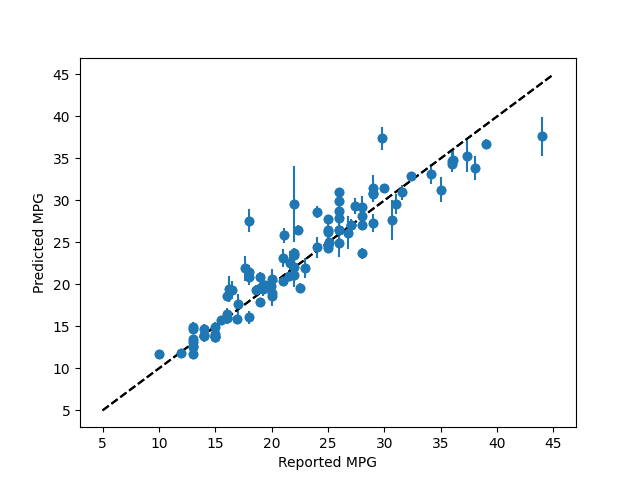# Plotting Regression Forest Error Bars¶

This example demonstrates using forestci to calculate the error bars of the predictions of a `sklearn.ensemble.RandomForestRegressor` object.

The data used here are a classical machine learning data-set, describing various features of different cars, and their MPG.```# Regression Forest Example
import numpy as np
from matplotlib import pyplot as plt
from sklearn.ensemble import RandomForestRegressor
import sklearn.model_selection as xval
from sklearn.datasets import fetch_openml
import forestci as fci

# retreive mpg data from machine learning library
mpg_data = fetch_openml(data_id=196)

# separate mpg data into predictors and outcome variable
mpg_X = mpg_data["data"]
mpg_y = mpg_data["target"]

# remove rows where the data is nan
not_null_sel = np.where(mpg_X.isna().sum(axis=1).values == 0)
mpg_X = mpg_X.values[not_null_sel]
mpg_y = mpg_y.values[not_null_sel]

# split mpg data into training and test set
mpg_X_train, mpg_X_test, mpg_y_train, mpg_y_test = xval.train_test_split(
mpg_X,
mpg_y,
test_size=0.25,
random_state=42)

# Create RandomForestRegressor
n_trees = 2000
mpg_forest = RandomForestRegressor(n_estimators=n_trees, random_state=42)
mpg_forest.fit(mpg_X_train, mpg_y_train)
mpg_y_hat = mpg_forest.predict(mpg_X_test)

# Plot predicted MPG without error bars
plt.scatter(mpg_y_test, mpg_y_hat)
plt.plot([5, 45], [5, 45], 'k--')
plt.xlabel('Reported MPG')
plt.ylabel('Predicted MPG')
plt.show()

# Calculate the variance
mpg_V_IJ_unbiased = fci.random_forest_error(mpg_forest, mpg_X_train,
mpg_X_test)

# Plot error bars for predicted MPG using unbiased variance
plt.errorbar(mpg_y_test, mpg_y_hat, yerr=np.sqrt(mpg_V_IJ_unbiased), fmt='o')
plt.plot([5, 45], [5, 45], 'k--')
plt.xlabel('Reported MPG')
plt.ylabel('Predicted MPG')
plt.show()
```

Total running time of the script: ( 0 minutes 9.053 seconds)

Gallery generated by Sphinx-Gallery